# How to denote absolute value(|a|) symbol in LaTeX?

In this tutorial, I will discuss a very important topic, how to denote the absolute value with the help of latex.

There are many ways to denote the absolute value in latex but here I will discuss the best method. So, the absolute value symbol adjusts dynamically with your equation. And the mathematical equation looks more beautiful.

Case1. The absolute value symbol is bound by two vertical bars (|). Then you will think that you can easily add vertical bars on both sides of any expressions without commands because the vertical bar is available on your computer keyboard.

\documentclass{article}
\begin{document}
$$|x|$$
\end{document}


Output :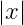Case2. Instead of typing a vertical bar on the keyboard, you can use the \mid command which will return you the same result.

\documentclass{article}
\begin{document}
$$\mid x \mid$$
\end{document}


Output :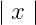If you look at the output above, using the \mid command increases the space on both sides of the x.

Case3. Third, you can use the \lvert and \rvert commands. And in this case, you need to type the expression between the two commands.

\documentclass{article}
\usepackage{amsmath}
\begin{document}
$$\lvert x \rvert$$
\end{document}

Output :In this case, there is no space on either side of x. And the output is like the first case.

And below we will create a problem by combining the above three cases. And I will try to understand the difference between them.

\documentclass{article}
\usepackage{amsmath}
\begin{document}
$$|\frac{\partial x}{\partial t}|$$
$$\mid\frac{\partial x}{\partial t}\mid$$
$$\lvert \frac{\partial x}{\partial t} \rvert$$
\end{document}


Output :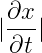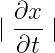If you look at this output, the vertical bars are two smaller according to the symbol of the partial time derivative. That is, the absolute value symbol does not match the size of the expression.

So, using vertical bars, \mid and \lvert..\rvert command is not the best practice. But, in the case of any single letter, that’s fine.

Also Read : How to write a vector in latex?

## Responsive absolute value symbol in latex

That is, you need to place a vertical bar on both sides of an expression in such a way that the vertical bar is responsive to the expression. For this you need to use the \left and \right commands on both sides of the vertical bar.

Suppose, I will solve the above problem. The problem is to represent the partial time derivative with absolute value, whose size matches dynamically.

\documentclass{article}
\begin{document}
$$\left|\frac{\partial x}{\partial t}\right|$$
\end{document}

Output :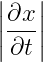Now, look at the output. In this case, both the vertical bar and the partial time derivative symbol are very nicely matched. And this is the best practice.

Always use the \left and \right commands before both vertical bars. However, you do not have to pass any argument with this command.

You can also use the \left and \right command before the \lvert and \rvert command to complete the same task that was used before the vertical bar.

\documentclass{article}
\usepackage{amsmath}
\begin{document}
$$\left \lvert \sum_{i=1}^{n} x_{i} \right \rvert$$
\end{document}

Output :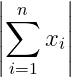Many times you will see that double vertical bars ||…|| are used on both sides of the matrix. The first two vertical bars denote the matrix and the second two vertical bars denote the value of the matrix. So, look at this example below

\documentclass{article}
\usepackage{amsmath}
\begin{document}
$$\left \lvert \begin{vmatrix} a & b\\ c & d \end{vmatrix} \right \rvert$$
\end{document}

Output :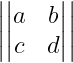You all know that the value of mod x always depends on the range of x. Many times mod is expressed by the absolute value symbol. So, look at this example below

\documentclass{article}
\usepackage{amsmath}
\begin{document}
$$\left | x \right |= \begin{cases} -x & \text{ if } x<0 \\ 0 & \text{ if } x=0 \\ x & \text{ if } x>0 \end{cases}$$
\end{document}

Output :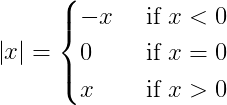## Conclusion

Hopefully, you have learned to use the latex absolute value symbol. You will always use \left and \right command with any vertical bar(|..|) or any other command for the absolute value symbol. As a result, the vertical bar will take shape according to the size of the expression.

It would be nice to see a written equation in your science document. And the size of the absolute value symbol will dynamically decrease and increase. Thank you